Also called Jacobi Quadrature or Mehler Quadrature. A Gaussian Quadrature over the intervalwith Weighting Function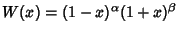. The Abscissas for quadrature orderare given by the roots of the Jacobi Polynomials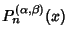. The weights are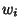(1)

whereis the Coefficient of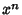in. For Jacobi Polynomials,(2)

whereis a Gamma Function. Additionally,(3)

so(4)(5)

where(6)

The error term is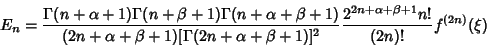(7)

(Hildebrand 1959).

References

Hildebrand, F. B. Introduction to Numerical Analysis. New York: McGraw-Hill, pp. 331-334, 1956.